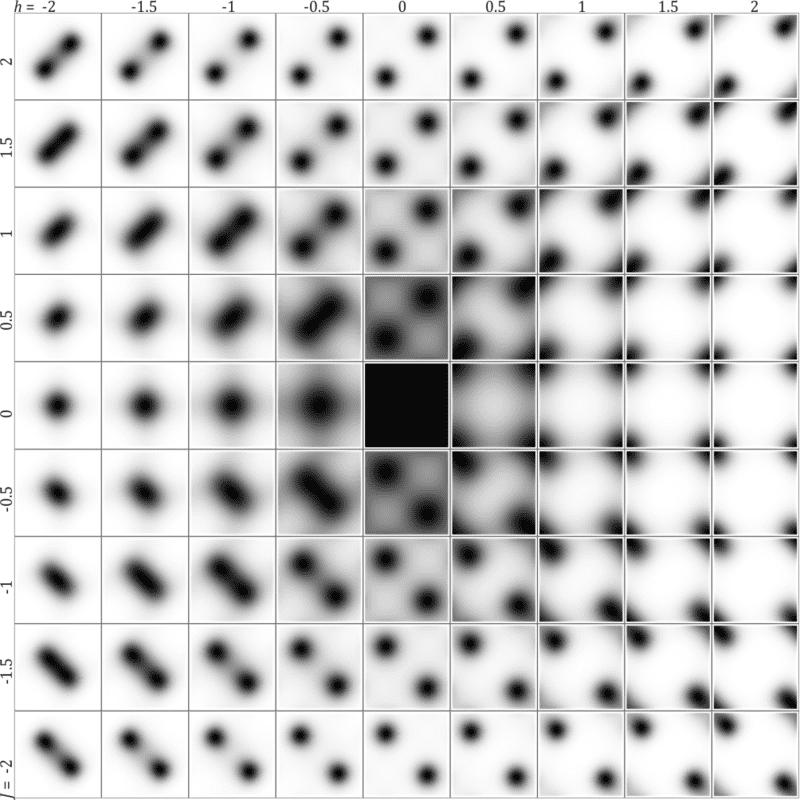# 1D transverse-field Ising model - classical vs quantum differences?

• A
Summary:
How to interpret intermediate spin angles in transverse-field Ising model? Should spin directions wobble thermally?
What are the differences between interpretations and predictions of classical and quantum treatment of this model?
The 1D transverse field Ising model
$$H(\sigma)=-J\sum_{i\in \mathbb{Z}} \sigma^x_i \sigma^x_{i+1} -h \sum_{i \in \mathbb{Z}} \sigma^z_i$$
is usually solved in quantum way, but we can also solve it classically - e.g. parametrize angles of spins ##\sigma^x_i = \cos(\alpha_i), \sigma^z_i=\sin(\alpha_i)## and use Boltzmann ensemble of sequences of spin angles:
$$\textrm{Pr}(\sigma)\propto \exp(-H(\sigma)) \qquad \textrm{for} \qquad \sigma = ((\cos(\alpha_i),\sin(\alpha_i)))_{i\in Z}$$
getting Markov process of angles, which can be easily approximated with Maximal Entropy Random Walk, for example leading to below joint distributions for ##(\alpha_i, \alpha_{i+1})\in [0,2\pi]^2## for various parameters (Section III here):As intuition suggests, there is some thermal wobbling of spin directions: (anti)aligned for dominating ##J##, in ##x## axis for dominating ##h##.

However, in quantum approaches there are only considered spins in four directions: ##|\leftarrow \rangle,|\rightarrow \rangle,|\uparrow \rangle,|\downarrow \rangle##, so should we imagine that intermediate angles are obtained by superposition?
Should there be thermal wobbling of spin directions as in densities above?

What are the differences in interpretation and predictions between such looking natural classical treatment and the quantum one?

Last edited: